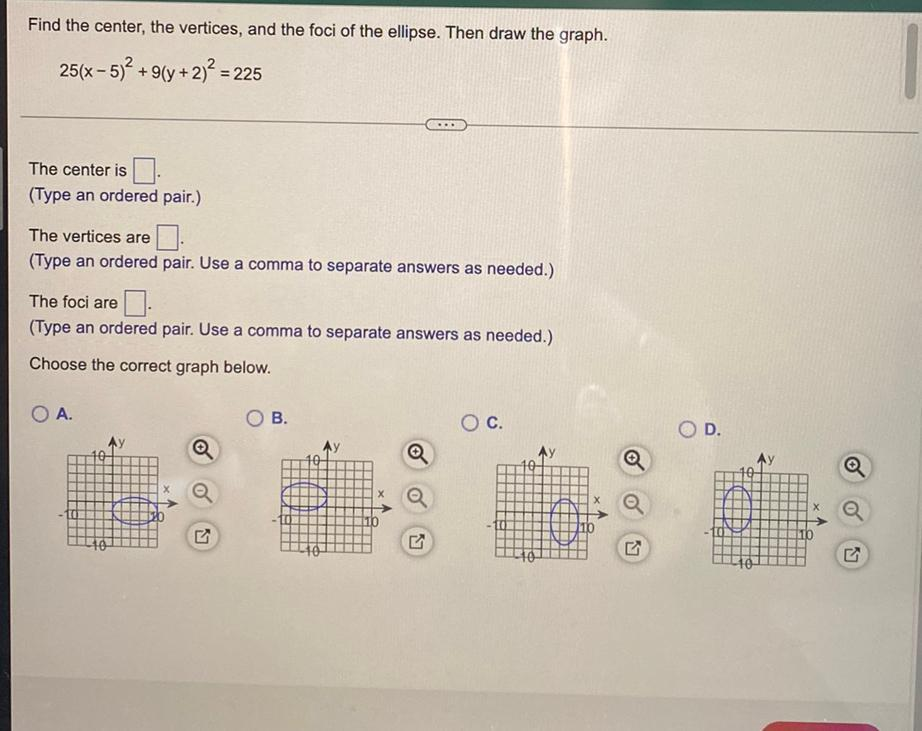Question:

# Find the center, the vertices, and the foci of the ellipse. Then draw the graph. 25(x - 5)2 +9(y + 2)2 = 225 + .. The center isFind the center, the vertices, and the foci of the ellipse. Then draw the graph. 25(x - 5)2 +9(y + 2)2 = 225 + .. The center is (Type an ordered pair.) The vertices are (Type an ordered pair. Use a comma to separate answers as needed.) The foci are (Type an ordered pair. Use a comma to separate answers as needed.) Choose the correct graph below. A. B. Oc. OD. a -100 LY 7 10